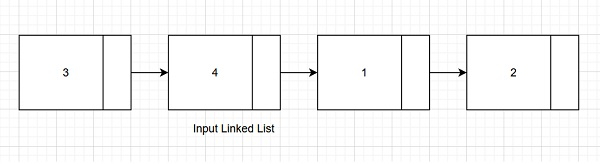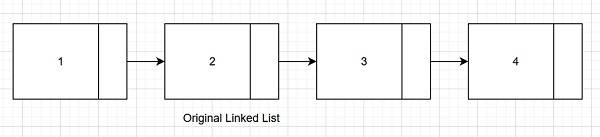# Count rotations in sorted and rotated linked list in C++

We are given a linked list. The list is sorted first and then rotated by K number of nodes. The goal is to find the value of K. If we are given below linked list as input which is rotated by K number of nodes −Then original must have been −And we can see K here is 2. Input linked list is a rotation of 2 nodes in the original sorted linked list.

Let us understand with examples.

Input − List : 5 → 7 → 9 → 1 → 3

Output

Elements in the linked list are: 5 7 9 1 3

Count of rotations in sorted and rotated linked list are − 3

Explanation − We can get the input list after three rotations in the original sorted list.

1 → 3 → 5 → 7 → 9, original
9 → 1 → 3 → 5 → 7, rotation 1
7 → 9 → 1 → 3 → 5, rotation 2
5 → 7 → 9 → 1 → 3 rotation 3

Input − List − 17 → 25 → 62 → 99

Output

Elements in the linked list are − 17 25 62 99

Count of rotations in sorted and rotated linked list are − 4

Explanation− We can get the input list after four rotations in the original sorted list.

17 → 25 → 62 → 99, original
99 → 17 → 25 → 62, rotation 1
62 → 99 → 17 → 25, rotation 2
25 → 62 → 99 → 17, rotation 3
17 → 25 → 62 → 99, rotation 4

## The approach used in the below program is as follows

The input Linked list would have one point at which the next node is smaller than the previous one. If the input string is also sorted then it is full-rotation of the original one. Starting from the head node, traverse till the (current node's data > head node’s data) and increment count. In case where (current node's data < head node’s data) break the loop. The count will have a number of rotations in the original list to get an input list.

• Take an input list and insert elements in it.

• Function insert_node(struct List_Node** head, int data) inserts nodes at the head of singly-linked lists with data.

• Function print(struct List_Node* node) prints the input Linked list starting from head till the end using while loop.

• Function rotations(struct List_Node* head) take the head pointer of the linked list and return the count of rotations done in the original linked list to get the input linked list.

• Take the initial count as 0.

• Take the temp variable as the data part of the head node.

• Using while loop, traverse till the end of the linked list is reached. (head!=NULL).

• Increment count in case every current node’s data is greater than temp.

• In case the current node’s data is less than the head node’s data ( temp). Break,

• We will count the number of rotations.

• Return count as result.

## Example

Live Demo

#include <bits/stdc++.h>
using namespace std;
struct List_Node{
int data;
struct List_Node* next;
};
int count = 0;
break;
}
count++;
}
return count;
}
void insert_node(struct List_Node** head, int data){
struct List_Node* new_node = new List_Node;
new_node->data = data;
}
void print(struct List_Node* node){
while (node != NULL){
cout<<node->data<<" ";
node = node->next;
}
}
int main(){
cout<<"Elements in the linked list are: ";
}
Elements in the linked list are: 28 35 18 1 2
Count of rotations in sorted and rotated linked list are: 2